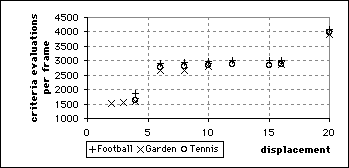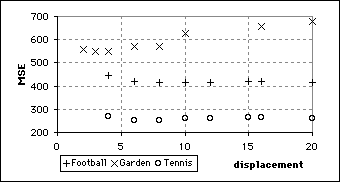## Greedy Algorithm D Results

The number of criteria evaluations required by Greedy Algorithm D appeared to be independent of the sequence coded. It also exhibited step behaviour but number of criteria evaluated stepped up at integral powers of 4 rather than 2. This was because Greedy Algorithm D quarters the stepsize rather than halves.Number of matching criteria evaluated by Greedy Algorithm D.MSE of sequences coded using Greedy Algorithm D with various maximum displacements.

This graph shows that the behaviour of the MSE for Greedy Algorithm D was very similar to that for Greedy Algorithm C, with low points at the same displacements. Algorithm D was more successful at coding with small displacements than Algorithm C however.